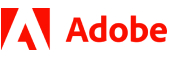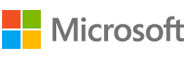New update is available. Click here to update.
Topics

# Count Inversions - ll

Moderate0/80
Average time to solve is 50m+1 more companies

## Problem statement

Given a Singly Linked List of integers, return its inversion count. The inversion count of a linked list is a measure of how far it is from being sorted.

Two nodes 'N1' and 'N2' form an inversion if the value of 'N1' is greater than the value of 'N2' and 'N1' appears before 'N2' in the linked list.

Detailed explanation ( Input/output format, Notes, Images )
Constraints:
``````0 <= L <= 10^5
-10^9 <= data <= 10^9 and data != -1

Where L is the number of nodes in the linked list and 'data' is the node value of the linked list.

Time Limit: 1 sec
``````
Sample Input 1:
``````3 2 1 5 4 -1
``````
Sample Output 1:
``````4
``````
Explanation of the Sample Input 1:
``````For the given linked list, there are 4 inversions: (3, 2), (3, 1), (2, 1) and (5, 4). Thus, the inversion count of the given linked list is 4.
``````
Sample Input 2:
``````0 6 8 7 -1
``````
Sample Output 2:
``````1
``````
Explanation of the Sample Output 2:
``````For the given linked list, there is only 1 inversion: (8, 7). Thus, the inversion count of the given linked list is 1.
``````Console